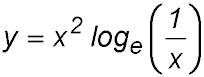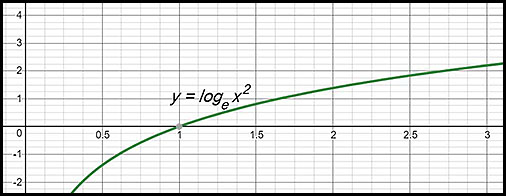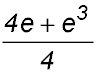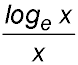Dr. J's Maths.com
Where the techniques of Maths
are explained in simple terms.

Functions - Logarithmic - Differentiation.
Applications - Test Yourself 1.

 The questions on this page focus on: determining gradients; equations of tangents and normals; maximum and minimum questions;

 Gradients 1. Show that the gradient of the curve y = loge (x2 + 1) is always positive. 2. Find the exact coordinates of the point on the curvewhere the gradient equals zero. Answer.At (1/√e, 1/(2e)). 3. Prove that the curve y = x2 + loge 2x can never have a stationary point. Tangents and normals 4. Find the equation of the tangent to y = 3 loge 4x at x = 3. Answer.y = x - 3 - 3ln 12. 5. Find the equation of the normal to y = loge (3x - 2) at the point (1, 0) in general form. Answer.x + 3y - 1 = 0. 6. Determine the equation of the tangent to the curve y = 2ln (2 - 5x2) at the point where x = 0. Answer.y = 2 ln 2 7.The function y = loge(x2) is graphed above for x > 0. (i) Show that the tangent to the curve at the point P (e, 2) passes through the origin O. (ii) Find the equation of the normal to the curve at P and then find the point Q where that normal meets the y-axis. (iii) Show that the area of the triangle OPQ isu2. 8. Find the equation of the tangent to the curve y = x2 ln x at the point where x = e. Answer.y = 3ex - 2e2 9. 10. Maximum and minimum questions 11. (i) Find the stationary point on the curve y = 4 ln (x2 + 1). (ii) For what values of x is the concavity of this curve always positive? Answer.(i) SP at (0, 0)(ii) Positive concavity: -1 < x < 1. 12. (i) Draw the graphs of y = ln(x - 1) and y = x on the same set of axes. (ii) If D represents the distance between the two curves, write down an expression for D. (iii) If X represents the value on the x-axis where this distance between the two curves is the shortest, find the value for X. (iii) Find the minimum distance between the two curves. Answer.(ii) X = 2(iii)D = 2.13. In the diagram, P is a point on the curve y = x2 - 4x + 7 Q is a point on the curve y = ln (x + 2) P and Q have the same x coordinates. Find the exact minimum distance for PQ between the two curves. Answer.Min PQ = 1.60 (2 dec places). 14. For what value of x is the value for the ratioa maximum? Answer.x = e. 15.